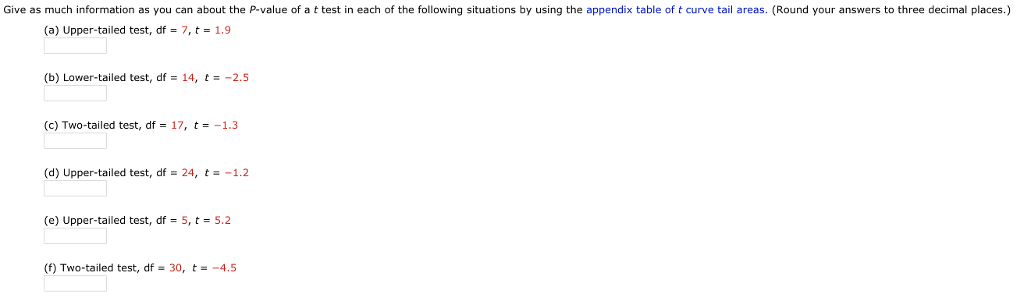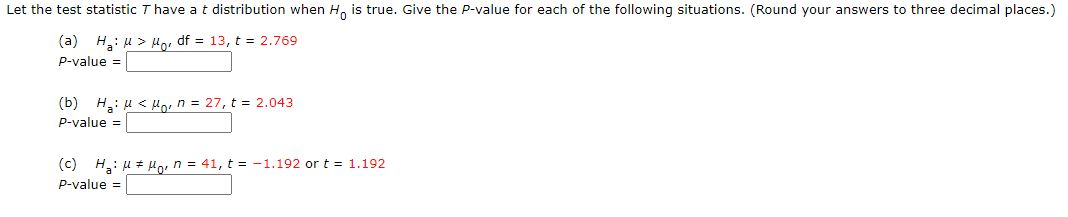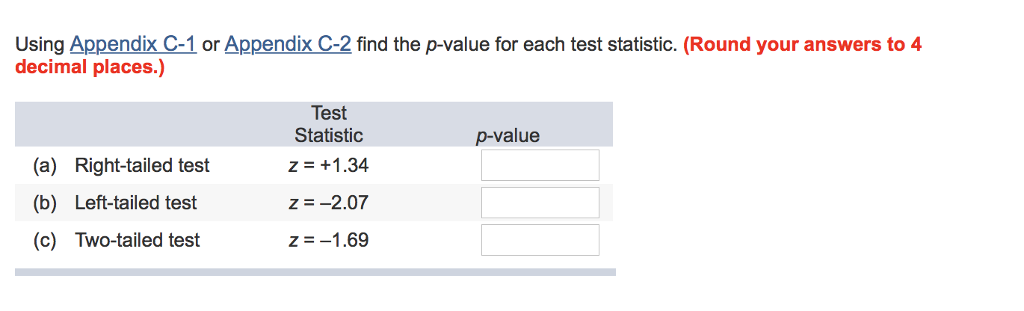Question

# For each statistic below, give the p value (do not round p): 1. t=2.20, df=15, one...

For each statistic below, give the p value (do not round p):

1. t=2.20, df=15, one tailed test

We have given,

One tailed test,

df=15

t =2.20

P value =0.021948................by using Excel =TDIST(ABS(2.2),15,1)

#### Earn Coins

Coins can be redeemed for fabulous gifts.

Similar Homework Help Questions
• ### For each test statistic below, give the p value (do not round p): a. t =...

For each test statistic below, give the p value (do not round p): a. t = 2.20, df = 15, one-tailed test b. Z = 1.10, one-tailed test c. t = -2.65, df = 20, two-tailed test d. Z = -.26, two-tailed test

• ### Give as much information as you can about the P-value of a t test in each...Give as much information as you can about the P-value of a t test in each of the following situations by using the appendix table of t curve tail areas. (Round your answers to three decimal places.) (a) Upper-tailed test, df-7,t-1.9 (b) Lower-tailed test, df- 14, t -2.5 (c) Two-tailed test, df- 17, t-1.3 (d) Upper-tailed test, df = 24, --1.2 (e) upper-tailed test, df = 5, t = 5.2 (f) Two-tailed test, df 30, t-4.5

• ### Give as much information as you can about the P-value of a t test in each...

Give as much information as you can about the P-value of a t test in each of the following situations. (Round your answers to three decimal places.) (a) Upper-tailed test, df = 9, t = 2.0 P-value < 0.005 0.005 < P-value < 0.01 0.01 < P-value < 0.025 0.025 < P-value < 0.05 P-value > 0.05 (b) Upper-tailed test, n = 13, t = 3.2 P-value < 0.005 0.005 < P-value < 0.01 0.01 < P-value < 0.025 0.025...

• ### Let the test statistic T have a t distribution when He is true. Give the P-value...Let the test statistic T have a t distribution when He is true. Give the P-value for each of the following situations. (Round your answers to three decimal places.) (a) Hu > Mor df = 13, t = 2.769 P-value = (b) Haru<llo, n = 27, t = 2.043 P-value = (c) Hiu * Mo, n = 41, t = -1.192 or t = 1.192 P-value =

• ### Using Appendix C-1 or Appendix C-2 find the p-value for each test statistic. (Round your answers...Using Appendix C-1 or Appendix C-2 find the p-value for each test statistic. (Round your answers to 4 decimal places.) Test Statistic z = +1.34 p-value (a) Right-tailed test (b) Left-tailed test (c) Two-tailed test z-_2.07 z =-1.69

• ### For each of the following t values (a-d), indicate whether the t statistic is statistically significant...

For each of the following t values (a-d), indicate whether the t statistic is statistically significant for a two-tailed test, at the specified alphas: t = 2.90, df = 25, α = 0.01 t = 2.20, df = 25, α = 0.05 t = 5.52, df = 10, α = 0.01 t = 2.02, df = 20, α = 0.05 CAUTION: GET ON THE RIGHT PAGE …..Make sure you use the correct table: pages 413-415 (the difference is the α:...

• ### What is the p-value of a two-tailed one-mean hypothesis test, with a test statistic of z0=−1.73?...

What is the p-value of a two-tailed one-mean hypothesis test, with a test statistic of z0=−1.73? (Do not round your answer; compute your answer using a value from the table below.) z−1.8−1.7−1.6−1.5−1.40.000.0360.0450.0550.0670.0810.010.0350.0440.0540.0660.0790.020.0340.0430.0530.0640.0780.030.0340.0420.0520.0630.0760.040.0330.0410.0510.0620.0750.050.0320.0400.0490.0610.0740.060.0310.0390.0480.0590.0720.070.0310.0380.0470.0580.0710.080.0300.0380.0460.0570.0690.090.0290.0370.0460.0560.068

• ### Use technology to find the​ P-value for a​ two-tailed test with n equals=15 and test statistic...

Use technology to find the​ P-value for a​ two-tailed test with n equals=15 and test statistic t equals negative t=−2.143.

• ### Use technology to find the​ P-value for a​ two-tailed test with nequals11 and test statistic t...

Use technology to find the​ P-value for a​ two-tailed test with nequals11 and test statistic t equals 3.075 . ​P-valuealmost equals nothing ​(Round to four decimal places as​ needed.)

• ### Assuming the actual test statistic is t = 2.10, with df = 10, what is the...

Assuming the actual test statistic is t = 2.10, with df = 10, what is the p-value for the test in part